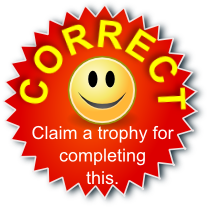# Laws of Indices - True or False?

##### Level 1Level 2Level 3Level 4Exam-StyleGameDescriptionHelpMore Indices

Arrange the given statements involving indices to show whether they are true or false.

## FALSE

$$(x^3)^4 \equiv x^7$$

$$\frac{x^6}{x^3} \equiv x^2$$

$$x^8 \div x^4 \equiv x^2$$

$$x^2 \times x^3 \equiv x^6$$

$$(x^3)^4 \equiv x^{12}$$

$$\frac{x^7}{x^3} \equiv x^4$$

$$x^8 \div x^5 \equiv x^3$$

$$x^2 \times x^3 \equiv x^5$$This is Laws of Indices - True or False? level 1. You can also try:
Level 2 Level 3 Level 4

There are also a set of printable cards for an offline version.

## Transum.org

This web site contains over a thousand free mathematical activities for teachers and pupils. Click here to go to the main page which links to all of the resources available.## More Activities:

Mathematicians are not the people who find Maths easy; they are the people who enjoy how mystifying, puzzling and hard it is. Are you a mathematician?

Comment recorded on the 24 May 'Starter of the Day' page by Ruth Seward, Hagley Park Sports College:

"Find the starters wonderful; students enjoy them and often want to use the idea generated by the starter in other parts of the lesson. Keep up the good work"

Comment recorded on the 5 April 'Starter of the Day' page by Mr Stoner, St George's College of Technology:

"This resource has made a great deal of difference to the standard of starters for all of our lessons. Thank you for being so creative and imaginative."

Each month a newsletter is published containing details of the new additions to the Transum website and a new puzzle of the month.

The newsletter is then duplicated as a podcast which is available on the major delivery networks. You can listen to the podcast while you are commuting, exercising or relaxing.

Transum breaking news is available on Twitter @Transum and if that's not enough there is also a Transum Facebook page.

#### Without LiftingCan you draw these diagrams without lifting your pencil from the paper? This is an interactive version of the traditional puzzle. Some diagrams are possible while others are not. What is the rule?

## Numeracy

"Numeracy is a proficiency which is developed mainly in Mathematics but also in other subjects. It is more than an ability to do basic arithmetic. It involves developing confidence and competence with numbers and measures. It requires understanding of the number system, a repertoire of mathematical techniques, and an inclination and ability to solve quantitative or spatial problems in a range of contexts. Numeracy also demands understanding of the ways in which data are gathered by counting and measuring, and presented in graphs, diagrams, charts and tables."

Secondary National Strategy, Mathematics at key stage 3

## Go Maths

Learning and understanding Mathematics, at every level, requires learner engagement. Mathematics is not a spectator sport. Sometimes traditional teaching fails to actively involve students. One way to address the problem is through the use of interactive activities and this web site provides many of those. The Go Maths main page links to more activities designed for students in upper Secondary/High school.

## Teachers

If you found this activity useful don't forget to record it in your scheme of work or learning management system. The short URL, ready to be copied and pasted, is as follows:

Do you have any comments? It is always useful to receive feedback and helps make this free resource even more useful for those learning Mathematics anywhere in the world. Click here to enter your comments.For All:

## Description of Levels

CloseLevel 1 - The basic laws of indices

Level 2 - More complex statements including negative indices

Level 3 - More complex statements including fractional indices

Level 4 - Mixed puzzling statements for the expert

Cards - There are also a set of printable cards for an offline version of this activity.

Game - The Indices Pairs game with three levels of difficulty.

Exam Style questions are in the style of GCSE or IB/A-level exam paper questions and worked solutions are available for Transum subscribers.

More on this topic including lesson Starters, visual aids and investigations.

Answers to this exercise are available lower down this page when you are logged in to your Transum account. If you don’t yet have a Transum subscription one can be very quickly set up if you are a teacher, tutor or parent.

## Curriculum Reference

See the National Curriculum page for links to related online activities and resources.

## Examples

 $$5^a \times 5^b \equiv 5^{a+b}$$ $$5^a \div 5^b \equiv 5^{a-b}$$ $$(5^a)^b \equiv 5^{ab}$$ $$5^1 \equiv 5$$ $$5^0 \equiv 1$$ $$5^{-1} \equiv \frac15$$ $$5^{-2} \equiv \frac{1}{25}$$ $$5^{\frac12} \equiv \sqrt{5}$$ $$5^{\frac13} \equiv \sqrt{5}$$ $$5^{\frac23} \equiv \sqrt{5^2}$$

Answers to this exercise are available lower down this page when you are logged in to your Transum account. If you don’t yet have a Transum subscription one can be very quickly set up if you are a teacher, tutor or parent.

Close